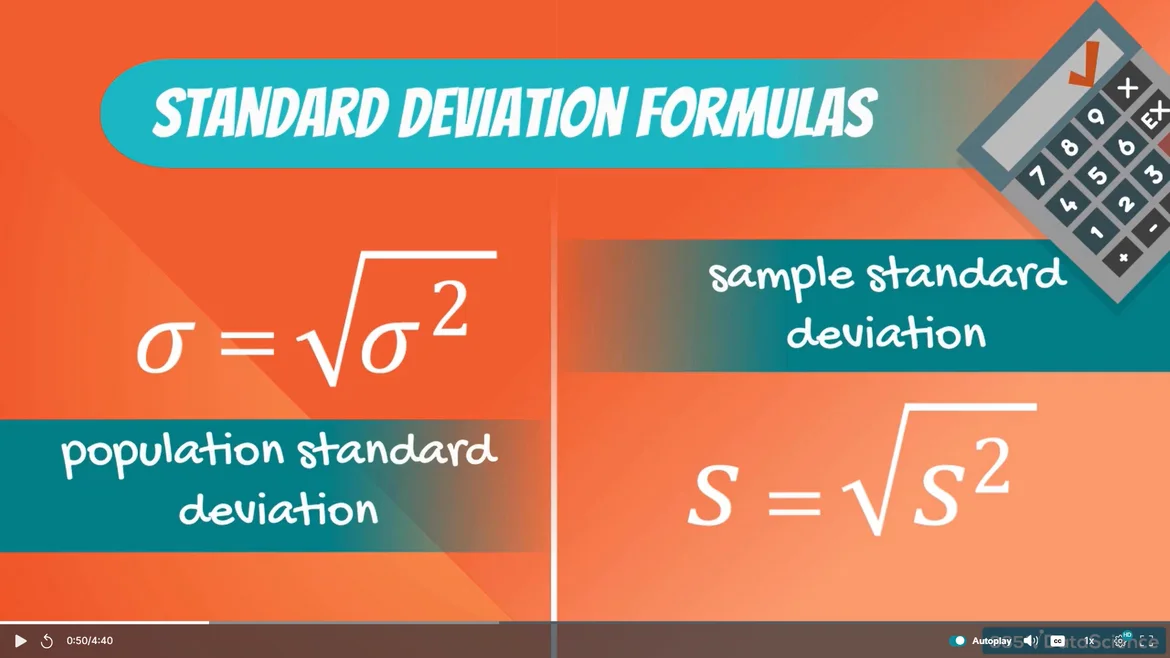Posted on:

27 Dec 2022

0

# (Resolved) Didn't understand why we even need standard deviation formulas same to variance

Resolved after getting back to variance lecture and finding that Variance actually Sigma Square not just sigma (don't know why we denote variance with Sigma square although)

I have attached an image of the lecture snapped at 00:50 of this lecture where
Population standard deviation  = Square root(variance*sqare)
same with the Sample formula if we apply math then both sides are exactly the same like Sqare RootandSqarecancel each other and
what we got is Standard deviation = Variance

sowhat's the need and importance remain when both have the same formulas